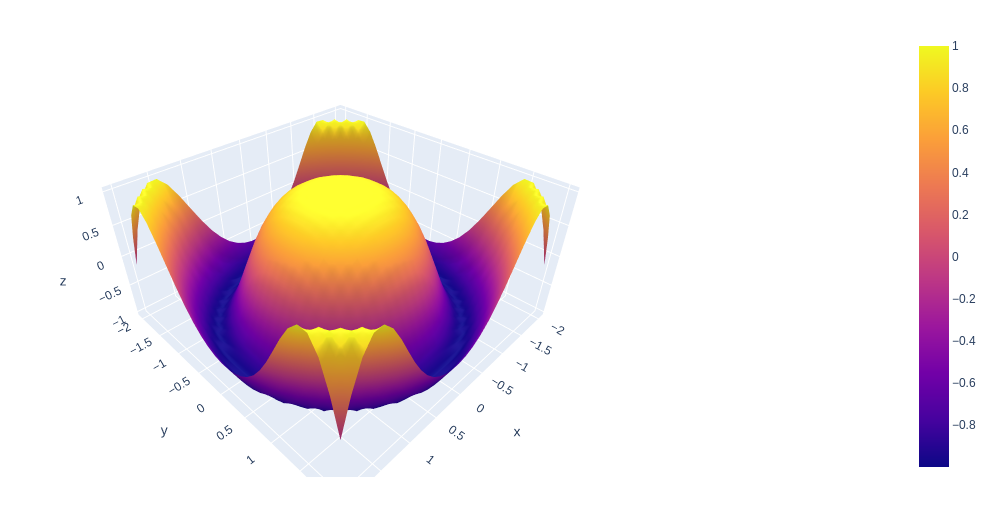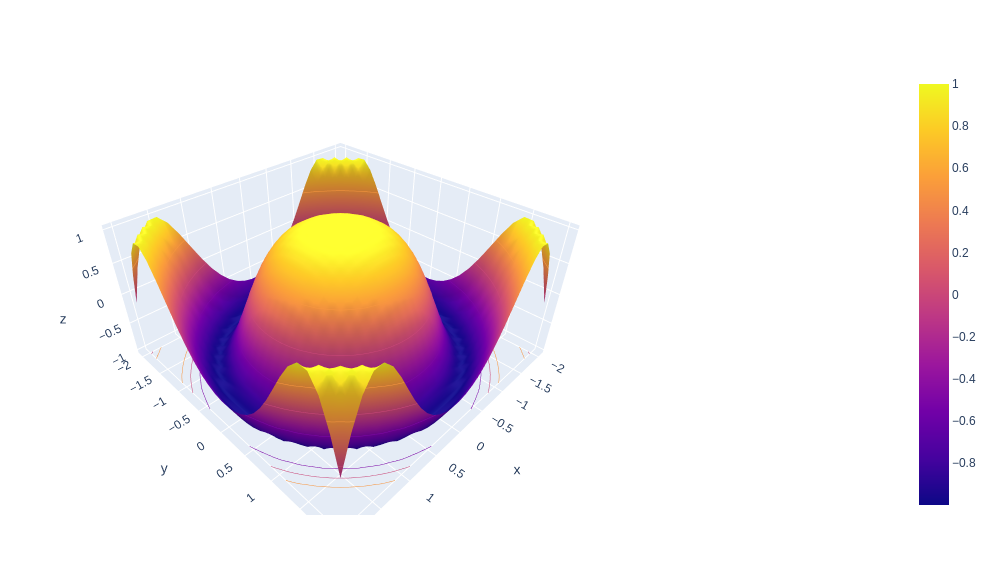GeeksforGeeks App
Open AppBrowser
Continue

# 3D Surface Plots using Plotly in Python

Plotly is a Python library that is used to design graphs, especially interactive graphs. It can plot various graphs and charts like histogram, barplot, boxplot, spreadplot, and many more. It is mainly used in data analysis as well as financial analysis. plotly is an interactive visualization library.

## Surface Plot plotly

Surface plot is those plot which has three-dimensions data which is X, Y, and Z. Rather than showing individual data points, the surface plot has a functional relationship between dependent variable Y and have two independent variables X and Z. This plot is used to distinguish between dependent and independent variables.

Syntax: plotly.graph_objects.Surface(arg=None, hoverinfo=None, x=None, y=None, z=None, **kwargs)

Parameters:

arg:  dict of properties compatible with this constructor or an instance of plotly.graph_objects.Surface

x: Sets the x coordinates.

y: Sets the y coordinates.

z: Sets the z coordinates.

hoverinfo: Determines which trace information appear on hover. If none or skip are set, no information is displayed upon hovering. But, if none is set, click and hover events are still fired.

Example:

## Python3

 `import` `plotly.graph_objects as go``import` `numpy as np`` ` `x ``=` `np.outer(np.linspace(``-``2``, ``2``, ``30``), np.ones(``30``))``y ``=` `x.copy().T``z ``=` `np.cos(x ``*``*` `2` `+` `y ``*``*` `2``)`` ` `fig ``=` `go.Figure(data``=``[go.Surface(x``=``x, y``=``y, z``=``z)])`` ` `fig.show()`

Output:## Showing Surface Plot With Contours

In plotly, contours attribute is used to Display and customize contour data for each axis.

Example:

## Python3

 `import` `plotly.graph_objects as go``import` `numpy as np`` ` `x ``=` `np.outer(np.linspace(``-``2``, ``2``, ``30``), np.ones(``30``))`` ` `# transpose``y ``=` `x.copy().T``z ``=` `np.cos(x ``*``*` `2` `+` `y ``*``*` `2``)`` ` `fig ``=` `go.Figure(data``=``[go.Surface(x``=``x, y``=``y, z``=``z)])`` ` `fig.update_traces(contours_z``=``dict``(``    ``show``=``True``, usecolormap``=``True``,``    ``highlightcolor``=``"limegreen"``,``    ``project_z``=``True``))`` ` `fig.show()`

Output:My Personal Notes arrow_drop_up